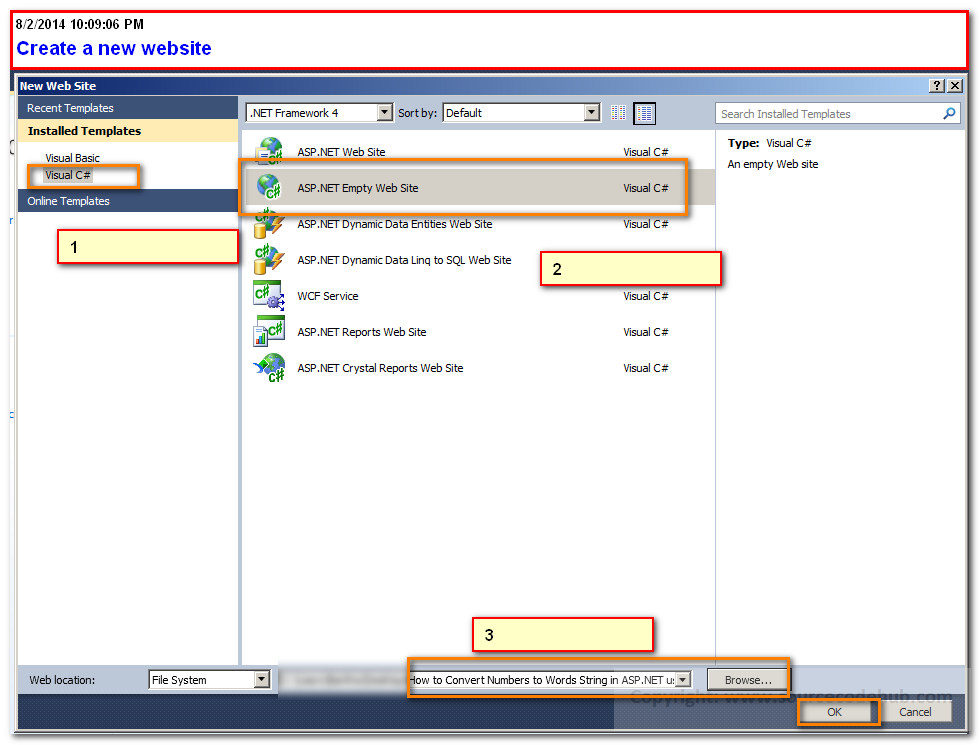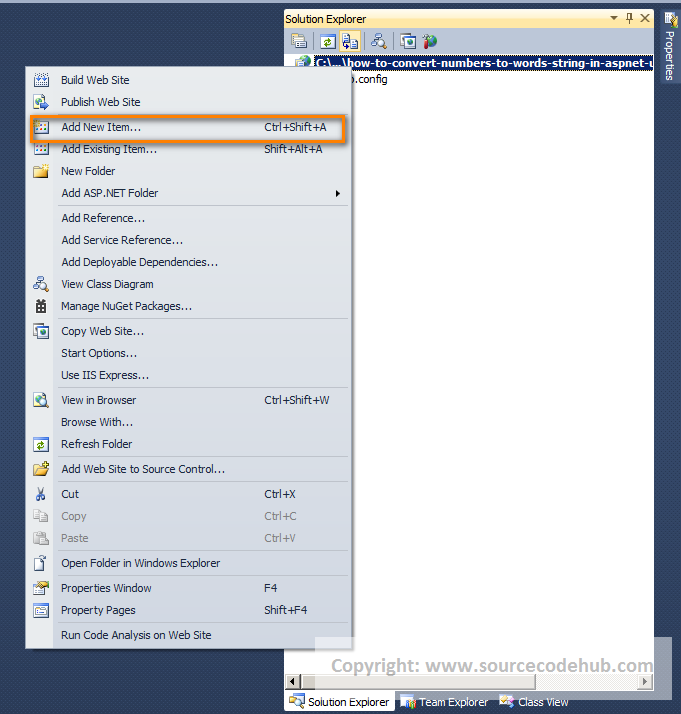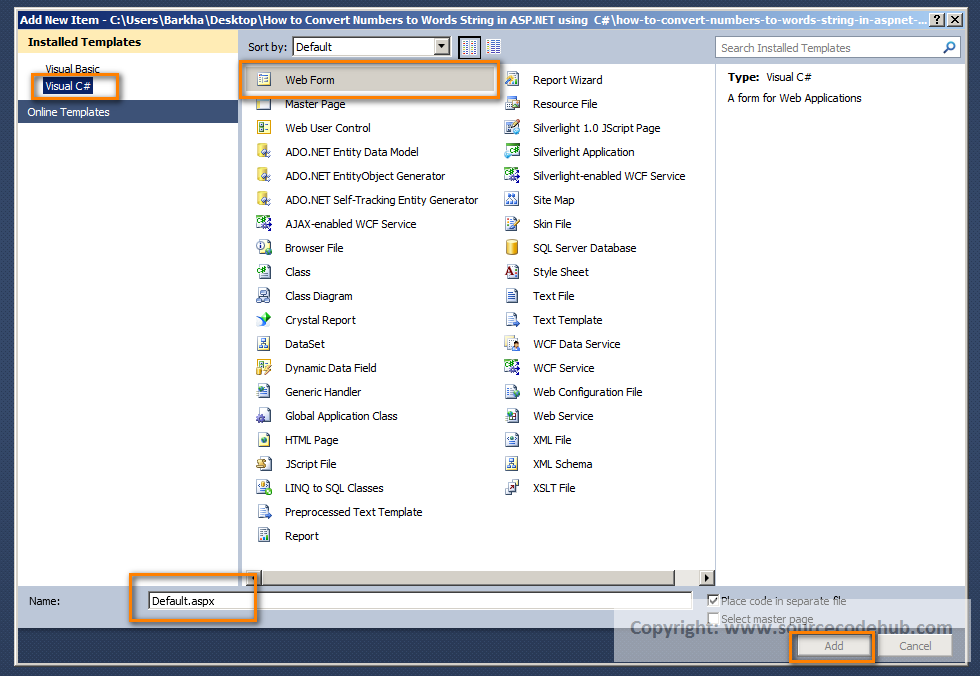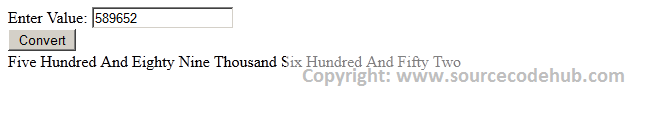# How to Convert Numbers to Words String in ASP.NET using C#

Category > CSHARP || Published on : Saturday, August 2, 2014 || Views: 15562 || Convert number to words Convert number to string C Sharp to convert a number to string ASP.NET Trick

Introduction:

Here Pawan Kumar will explain how to convert numbers to words in asp.net using c# with detailed example. In some condtion we need to convert number to string words

Description:

In previous post I explained How to Send Bulk Email in Asp.net with Example using C# ,How can we call a C# Method from ASPX Page or Code Behind page,How to validate or check whether checkbox is checked or not using ASP.NET and jQuery with example. ,Set meta tag in asp.net programmaticaly for creating seo friendly websites many more articles related to asp.net using c#, vb.net. Now I will explain how to convert numbers to words in asp.net using c# with detailed example and you can download the complete source code in asp.net and C Sharp

To convert numbers to string in asp.net using c#. We have to follow the following procedure

Step 1: Create a new ASP.NET empty Website using Visual Studio 2010. You can download the Visual Studio hereStep 2: Create a new ASPX page by right clicking on the Solution Explorer or from the File Menu and made the neccessary changes as below.```
<%@ Page Language="C#" AutoEventWireup="true" CodeFile="Default.aspx.cs" Inherits="_Default" %>

```

how-to-convert-numbers-to-words-string-in-aspnet-using-csharp-step1

Enter Value:

Step 3 : after that Press F7 and write the following C# Codes in the Code Behind.

```
using System;

using System.Collections.Generic;

using System.Linq;

using System.Web;

using System.Web.UI;

using System.Web.UI.WebControls;

public partial class _Default : System.Web.UI.Page

{

protected void Page_Load(object sender, EventArgs e)

{

}

protected void btnClick_Click(object sender, EventArgs e)

{

string word = ConvertNumbertoWords(Convert.ToInt32(tbNumber.Text));

lblMsg.InnerText = word;

}

public static string ConvertNumbertoWords(int number)

{

if (number == 0)

{

return "ZERO";

}

if (number < 0)

{

return "minus " + ConvertNumbertoWords(Math.Abs(number));

}

string strWords = "";

if ((number / 1000000) > 0)

{

strWords += ConvertNumbertoWords(number / 1000000) + " Million ";

number %= 1000000;

}

if ((number / 1000) > 0)

{

strWords += ConvertNumbertoWords(number / 1000) + " Thousand ";

number %= 1000;

}

if ((number / 100) > 0)

{

strWords += ConvertNumbertoWords(number / 100) + " Hundred ";

number %= 100;

}

if (number > 0)

{

if (strWords != "")

strWords += "And ";

var unitsMap = new[] { "Zero", "One", "Two", "Three", "Four", "Five", "Six", "Seven", "Eight", "Nine", "Ten", "Eleven", "Twelve", "Thirteen", "Fourteen", "Fifteen",

"Sixteen", "Sseventeen", "Eighteen", "Nineteen" };

var TensMap = new[] { "Zero", "Ten", "Twenty", "Thirty", "Forty", "Fifty", "Sixty", "Seventy", "Eighty", "Ninety" };

if (number < 20)

strWords += unitsMap[number];

else

{

strWords += TensMap[number / 10];

if ((number % 10) > 0)

strWords += " " + unitsMap[number % 10];

}

}

return strWords;

}

}

```

Step 5: Run the apllication, by pressing the F5 shortcut key on the Keyboard and write any number like 1234 in the textbox, We will get the following outputIn this article, we have learned,  How to Convert Numbers to Words String in ASP.NET using  C#